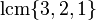# Element structure of symmetric group:S6

## Contents

This article gives specific information, namely, element structure, about a particular group, namely: symmetric group:S6.
View element structure of particular groups | View other specific information about symmetric group:S6

This article describes the element structure of symmetric group:S6.

For convenience, we take the underlying set to be$\{ 1,2,3,4,5,6\}$.

This group is NOT isomorphic to projective general linear group:PGL(2,9). For proof of the non-isomorphism, see PGL(2,9) is not isomorphic to S6. For the element structure of that group, see element structure of projective general linear group:PGL(2,9).

## Conjugacy class structure

FACTS TO CHECK AGAINST FOR CONJUGACY CLASS SIZES AND STRUCTURE:
Divisibility facts: size of conjugacy class divides order of group | size of conjugacy class divides index of center | size of conjugacy class equals index of centralizer
Bounding facts: size of conjugacy class is bounded by order of derived subgroup
Counting facts: number of conjugacy classes equals number of irreducible representations | class equation of a group

### Interpretation as symmetric group

FACTS TO CHECK AGAINST SPECIFICALLY FOR SYMMETRIC GROUPS AND ALTERNATING GROUPS:
Please read element structure of symmetric groups for a summary description.
Conjugacy class parametrization: cycle type determines conjugacy class (in symmetric group)
Conjugacy class sizes: conjugacy class size formula in symmetric group
Other facts: even permutation (definition) -- the alternating group is the set of even permutations | splitting criterion for conjugacy classes in the alternating group (from symmetric group)| criterion for element of alternating group to be real

For a symmetric group, cycle type determines conjugacy class, so the conjugacy classes are parametrized by the set of unordered integer partitions of the number 6.

Partition Partition in grouped form Verbal description of cycle type Representative element Size of conjugacy class Formula for size Even or odd? If even, splits? If splits, real in alternating group? Element orders Formula calculating element order
1 + 1 + 1 + 1 + 1 + 1 1 (6 times) six fixed points$()$ -- the identity element 1$\! \frac{6!}{(1)^6(6!)}$ even; no 1$\operatorname{lcm}\{ 1 \}$
2 + 1 + 1 + 1 + 1 2 (1 time), 1 (4 times) transposition, four fixed points$(1,2)$ 15$\! \frac{6!}{(2)(1)^4(4!)}$ odd 2$\operatorname{lcm}\{ 2, 1 \}$
3 + 1 + 1 + 1 3 (1 time), 1 (3 times) one 3-cycle, three fixed points$(1,2,3)$ 40$\! \frac{6!}{(3)(1)^3(3!)}$ even; no 3$\operatorname{lcm}\{ 3,1 \}$
4 + 1 + 1 4 (1 time), 1 (2 times) one 4-cycle, two fixed points$(1,2,3,4)$ 90$\! \frac{6!}{(4)(1)^2(2!)}$ odd 4$\operatorname{lcm}\{4,1 \}$
2 + 2 + 1 + 1 2 (2 times), 1 (2 times) double transposition: two 2-cycles, two fixed points$(1,2)(3,4)$ 45$\frac{6!}{(2)^2(2!)(1)^2(2!)}$ even; no 2$\operatorname{lcm}\{ 2, 1 \}$
5 + 1 5 (1 time), 1 (1 time) one 5-cycle, one fixed point$(1,2,3,4,5)$ 144$\! \frac{6!}{(5)(1)}$ even; yes; yes 5$\operatorname{lcm} \{ 5, 1 \}$
3 + 2 + 1 3 (1 time), 2 (1 time), 1 (1 time) one 3-cycle, one 2-cycle, one fixed point$(1,2,3)(4,5)$ 120$\! \frac{6!}{(3)(2)(1)}$ odd 6$\operatorname{lcm}\{3,2,1 \}$
2 + 2 + 2 2 (3 times) triple transposition$(1,2)(3,4)(5,6)$ 15$\! \frac{6!}{(2)^3(3!)}$ odd 2$\operatorname{lcm} \{ 2 \}$
4 + 2 4 (1 time), 2 (1 time) one 4-cycle, one 2-cycle$(1,2,3,4)(5,6)$ 90$\! \frac{6!}{(4)(2)}$ even; no 4$\operatorname{lcm} \{ 4 , 2 \}$
3 + 3 3 (2 times) two 3-cycles$(1,2,3)(4,5,6)$ 40$\! \frac{6!}{(3)^2(2!)}$ even; no 3$\operatorname{lcm} \{ 3 \}$
6 6 (1 time) one 6-cycle$(1,2,3,4,5,6)$ 120$\! \frac{6!}{6}$ odd 6$\operatorname{lcm} \{ 6 \}$
Total (11 rows = 11 conjugacy classes) -- -- -- 720 (equals order of the whole group) -- odd: 360, 5 classes
even;no: 216, 5 classes
even;yes;yes: 144, 1 class
order 1: 1, order 2: 75, order 3: 80, order 4: 180, order 5: 144, order 6: 240 --

## Automorphism class structure

Partitions for cycle types in one automorphism class Representative elements for each Size of each conjugacy class Number of classes Total size Element orders
1 + 1 + 1 + 1 + 1 + 1$()$ 1 1 1 1
2 + 1 + 1 + 1 + 1, 2 + 2 + 2$(1,2)$,$(1,2)(3,4)(5,6)$ 15 2 30 2
3 + 1 + 1 + 1, 3 + 3$(1,2,3)$,$(1,2,3)(4,5,6)$ 40 2 80 3
4 + 1 + 1$(1,2,3,4)$ 90 1 90 4
4 + 2$(1,2,3,4)(5,6)$ 90 1 90 4
5 + 1$(1,2,3,4,5)$ 144 1 144 5
3 + 2 + 1, 6$(1,2,3)(4,5)$,$(1,2,3,4,5,6)$ 120 2 240 6
2 + 2 + 1 + 1$(1,2)(3,4)$ 45 1 45 2
Total (8 classes) -- -- 11 720 --